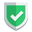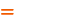我们相信：世界是美好的，你是我也是。平行空间的世界里面，不同版本的生活也在继续...

## 定位成员方式误区

• 比如读写二维数组的时候，就是通过`[][]`进行定位的（写了两个`[]`），这个是常识。
• 改成`[:]`的时候，就 也是通过`[:][:]`进行定位的，（注意是两个`[:]`

## 传统的[]定位成员

``````import numpy as np

s1 = np.array([["苏", "南", "大", "叔"], ["北", "京", "大", "学"], ["技", "术", "经", "理"]])
# s1 = [["苏", "南", "大", "叔"], ["北", "京", "大", "学"], ["技", "术", "经", "理"]]
print(s1)             # ['苏' '南' '大' '叔']
print(s1)          # 苏
print(s1)             # ['北' '京' '大' '学']
print(s1)          # 京
print(s1[0,0])           # 苏
print(s1[1,1])           # 京

s1 = np.arange(27).reshape(-1,3,3)
print(s1.shape)
print(s1[0,0,0])          # 0
print(s1[1,2,1])          # 16``````

## 使用[:]定位成员

``````import numpy as np

s1 = np.array([["苏", "南", "大", "叔"], ["北", "京", "大", "学"], ["技", "术", "经", "理"]])
# s1 = [["苏", "南", "大", "叔"], ["北", "京", "大", "学"], ["技", "术", "经", "理"]]
print(s1[0:2])             # [['苏' '南' '大' '叔'] ['北' '京' '大' '学']]
print(s1[0:2])          # ['北' '京' '大' '学']
print(s1[0:2][1:2])        # [['北' '京' '大' '学']]
print(s1[0:2][1:2])     # ['北' '京' '大' '学']
print(s1[0:2][1:2])  # 京``````
`s1[0:2][1:2]``[1:2]`是在`[0:2]`里面再次挑选。

## 再解释一次

• `[:]`操作，一直保持原维度不变，并且有容错性，超限不报错。
• `[]`操作，会降低到下一个维度，维度减一，超出索引值报错。

``````import numpy as np
s1 = np.array([["苏", "南", "大", "叔"], ["北", "京", "大", "学"], ["技", "术", "经", "理"]])
print(s1[0:2][8:9])        # 不报错，返回[]
print(s1[0:2][1:2])     # 报错``````

``````[]
Traceback (most recent call last):
File "C:\Users\sunan\Desktop\demo\py\t.py", line 4, in <module>
print(s1[0:2][1:2])     # 报错
IndexError: index 9 is out of bounds for axis 0 with size 1``````

## 结束语

`python`是一条大蟒蛇，掌握它的习性需要付出不断的尝试和努力。如果本文对您有帮助，或者节约了您的时间，欢迎打赏瓶饮料，建立下友谊关系。本博客不欢迎：各种镜像采集行为。请尊重原创文章内容，转载请保留作者链接。【福利】 腾讯云最新爆款活动！1核2G云服务器首年50元！【源码】本文代码片段及相关软件，请点此获取更多信息【绝密】秘籍文章入口，仅传授于有缘之人python